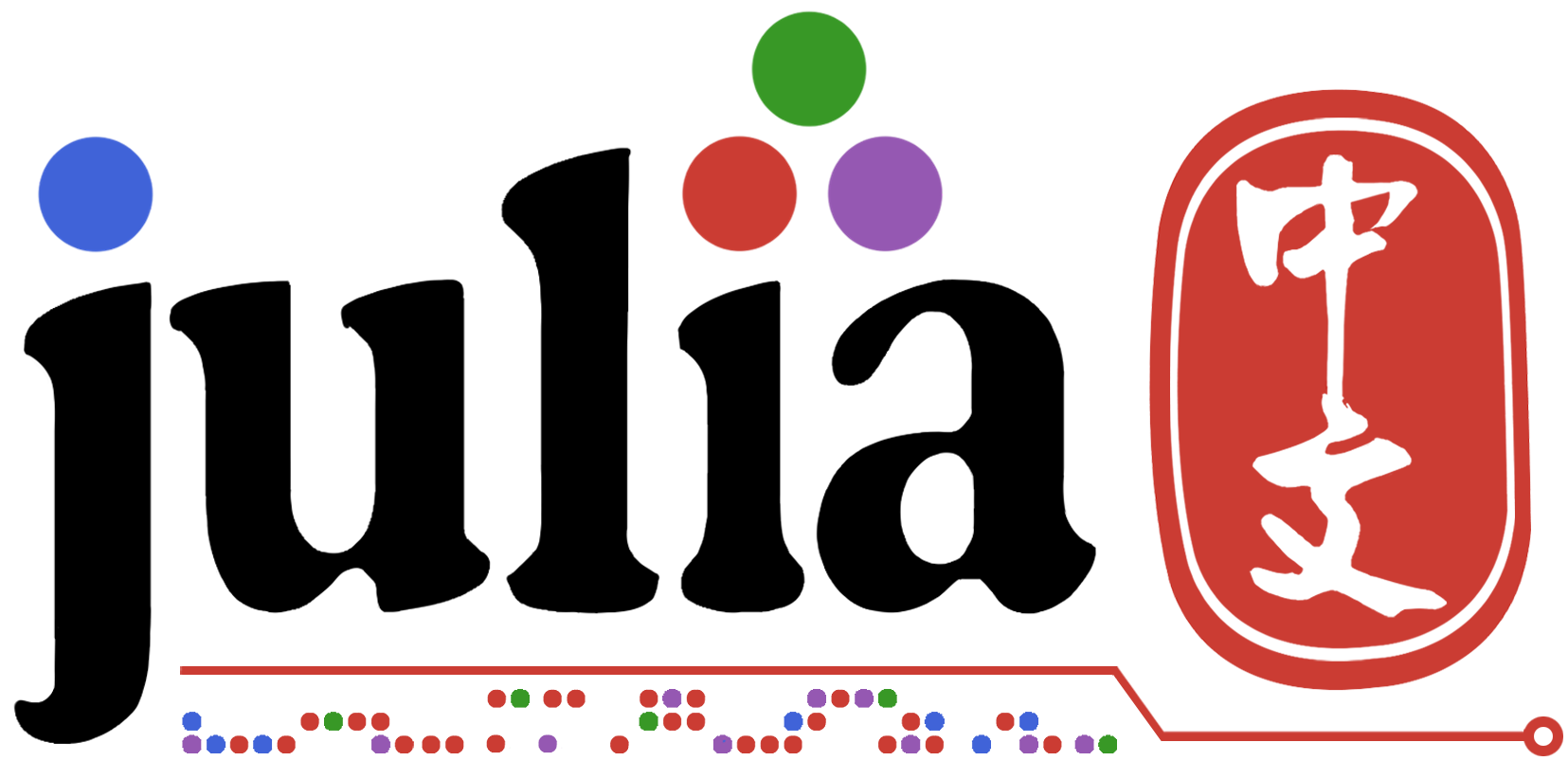1 个赞

(我把每一段都写得比较短, 感觉这样更容易读. 我一般把多余的信息写在小括号里, 不知道这样好不好. 如果可能的话, 希望可以在下方留些建议.)

# 但是!

``````temp1 = A + B
temp2 = temp1 + C
X = temp2 + D
``````

``````@assert ...
X = similar(D)
@inbounds for i in eachindex(X)
X[i] = A[i] + B[i] + C[i] + D[i]
end
``````

Julia的broadcast机制就是把 `D = A .+ B .+ C .+ D` 重新写成 `for` 循环. 所以说, 文档写了避免矢量化 (因为会造成没必要的内存浪费) 用broadcast.

# 总结

Julia的broadcast虽然看起来像MATLAB的风格的矢量化, 但从本质上不一样. Julia用的 `for` 循环, MATLAB的矢量化是调用别的库. 而且, Julia的broadcast十分灵活而且很容易拓展, 甚至可以放在GPU. 所以, 把broadcast想成一个对循环的抽象就好. 虽然感觉还有很多东西没有提到, 但是我感觉这么多也很够了. 是否要移到新的帖子上? @Gnimuc

# 脚注

``````julia> StridedArray
Union{DenseArray{T,N}, ReinterpretArray{T,N,S,A} where S where A<:Union{SubArray{T,N,A,I,true} where I<:Tuple{AbstractUnitRange,Vararg{Any,N} where N} where A<:DenseArray where N where T, DenseArray}, ReshapedArray{T,N,A,MI} where MI<:Tuple{Vararg{SignedMultiplicativeInverse{Int64},N} where N} where A<:Union{ReinterpretArray{T,N,S,A} where S where A<:Union{SubArray{T,N,A,I,true} where I<:Tuple{AbstractUnitRange,Vararg{Any,N} where N} where A<:DenseArray where N where T, DenseArray} where N where T, SubArray{T,N,A,I,true} where I<:Tuple{AbstractUnitRange,Vararg{Any,N} where N} where A<:DenseArray where N where T, DenseArray}, SubArray{T,N,A,I,L} where L where I<:Tuple{Vararg{Union{Int64, AbstractRange{Int64}, AbstractCartesianIndex},N} where N} where A<:Union{ReinterpretArray{T,N,S,A} where S where A<:Union{SubArray{T,N,A,I,true} where I<:Tuple{AbstractUnitRange,Vararg{Any,N} where N} where A<:DenseArray where N where T, DenseArray} where N where T, ReshapedArray{T,N,A,MI} where MI<:Tuple{Vararg{SignedMultiplicativeInverse{Int64},N} where N} where A<:Union{ReinterpretArray{T,N,S,A} where S where A<:Union{SubArray{T,N,A,I,true} where I<:Tuple{AbstractUnitRange,Vararg{Any,N} where N} where A<:DenseArray where N where T, DenseArray} where N where T, SubArray{T,N,A,I,true} where I<:Tuple{AbstractUnitRange,Vararg{Any,N} where N} where A<:DenseArray where N where T, DenseArray} where N where T, DenseArray}} where N where T
``````
5 个赞

1 个赞